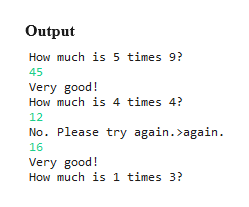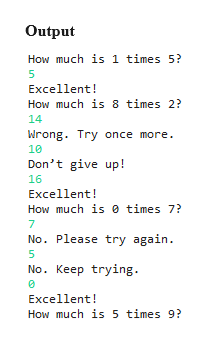Question
40 views

Preffered language is java. Will be compiled with intellij

Problem 1 consists of multiple parts. You should finish one part and ensure that it works before moving to the next part. You will need to refactor (rewrite parts of) your code as you move between parts.

#### Part 1

The use of computers in education is referred to as computer-assisted instruction (CAI). Write a program that will help an elementary school student learn multiplication. Use a SecureRandom object to produce two positive one-digit integers (you will need to look up how to do this). The program should then prompt the user with a question, such as

How much is 6 times 7?

The student then inputs the answer. Next, the program checks the student’s answer. If it’s correct, display the message "Very good!" and ask another multiplication question. If the answer is wrong, display the message "No. Please try again.>again." and let the student try the same question repeatedly until the student finally gets it right. A separate method should be used to generate each new question. This method should be called once when the application begins execution and each time the user answers the question correctly.

#### Part 2

Modify the program from Part 1 so that various comments are displayed for each answer as follows:

Possible responses to a correct answer:

Very good! Excellent! Nice work! Keep up the good work!

Possible responses to an incorrect answer:

No. Please try again. Wrong. Try once more. Don’t give up! No. Keep trying.

Use random-number generation to choose a number from 1 to 4 that will be used to select one of the four appropriate responses to each correct or incorrect answer. Use a switch statement to issue the responses.

#### Part 3

Modify the program from Part 2 to use your question generation method to ask the student 10 different questions. Give students only one chance at answering each question. Count the number of correct and incorrect responses typed by the student. After the program has asked 10 questions, and the student types 10 answers, your program should calculate the percentage that are correct. If the percentage is lower than 75%, display "Please ask your teacher for extra help.", then reset the program so another student can try it. If the percentage is 75% or higher, display "Congratulations, you are ready to go to the next level!", then reset the program so another student can try it.

#### Part 4

Modify the program from Part 3 to allow the user to enter a difficulty level. At a difficulty level of 1, the program should use only single-digit numbers in the problems; at a difficulty level of 2, numbers as large as two digits, and so on. Allow for four levels of difficulty.

#### Part 5

Modify the program from Part 4 to allow the user to pick a type of arithmetic problem to study. An option of 1 means addition problems only, 2 means multiplication problems only, 3 means subtraction problems only, 4 means division problems only and 5 means a random mixture of all these types.

check_circle

Step 1

Since there are multiple questions and you have not specified which part needs to be answered, we have done first three parts for you. If you want any other part to be answered, then repost the question again specifying the part number.

Step 2

Source Code for Part 1

import java.security.SecureRandom;

import java.util.Scanner;

public class Multiplication

{

public static int question(){

SecureRandom random = new SecureRandom();

int a = random.nextInt(10);

int b = random.nextInt(10);

System.out.println("How much is "+ a +  " times "+ b +  "?");

return a*b;

}

public static void main(String args[])

{

Scanner sc = new Scanner(System.in);

int input,number;

number = question();

while(true){

input = sc.nextInt();

if(input==number){

System.out.println("Very good!");

number = question();

}else{

}

}

}

}help_outlineImage TranscriptioncloseOutput How much is 5 times 9? 45 Very good! How much is 4 times 4? 12 No. Please try again. again. 16 Very good! How much is 1 times 3? fullscreen
Step 3

Source code for Part 2

import java.security.SecureRandom;

import java.util.Random;

import java.util.Scanner;

public class Multiplication_1

{

public static int question(){

SecureRandom random = new SecureRandom();

int a = random.nextInt(10);

int b = random.nextInt(10);

System.out.println("How much is "+ a +  " times "+ b +  "?");

return a*b;

}

public static void main(String args[])

{

Scanner sc = new Scanner(System.in);

int input,number;

number = question();

while(true){

input = sc.nextInt();

Random random = new Random();

int x = random.nextInt(4)+1;

if(input==number){

switch(x){

case 1:

System.out.println("Very good!");

break;

case 2:

System.out.println("Excellent!");

break;

case 3:

System.out.println("Nice work!");

break;

case 4:

System.out.println("Keep up the good ...help_outlineImage TranscriptioncloseOutput How much is 1 times 5? 5 Excellent! How much is 8 times 2? 14 Wrong. Try once more 10 Don't give up! 16 Excellent! How much is times 7? 7 No. Please try again. 5 No. Keep trying Excellent! How much is 5 times 9? fullscreen

### Want to see the full answer?

See Solution

#### Want to see this answer and more?

Solutions are written by subject experts who are available 24/7. Questions are typically answered within 1 hour.*

See Solution
*Response times may vary by subject and question.
Tagged in

### Java